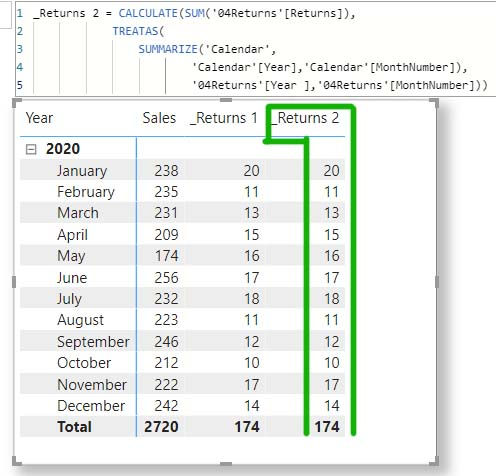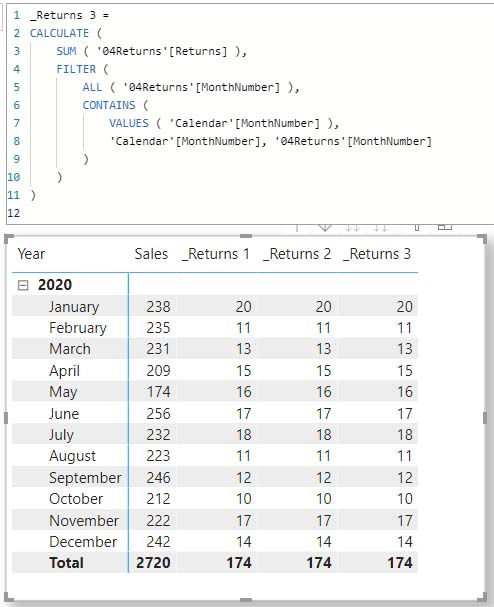top of page
Search
• Marek Vavrovic

# TREATAS

The TREATAS function applies the result of a table expression as a filter to the columns of an unrelated table. In other words, you can use it to apply filters to a table while there is no relationship present. In a normal scenario, tables are related to each other and when one table is filtered, the filter will propagate using the relationship. With TREATAS, we can filter unrelated tables, which makes it a really useful function.

Syntax:

TREATAS(table_expression, <column>[, <column>[, <column>[,…]]]} )

Notes:

• The number of columns specified must match the number of columns in the table expression and be in the same order.

• Best for use when a relationship does not exist between the tables. If you have multiple relationships between the tables involved, consider using USERELATIONSHIP instead.

• This function is not supported for use in DirectQuery mode when used in calculated columns or row-level security (RLS) rules.

Data model and source tables

I will be working with 3 tables. Calendar contains the dates, 03Sales has dates granularity and 04Returns has month granularity. Tables 03Sales and 04Returns are unrelated tables, means no relationship between them and filter propagation is not working.If I drag the Returns column (from 04Returns) into the table in the middle, together with the Sales column (from 03Sales) I will get back an incorrect result, the same total number for each row in the table.Solution 1

TREATAS with VALUES function

syntax

VALUES(<TableNameOrColumnName>)

_Returns 1 =

CALCULATE (

(<target_measure>),

TREATAS (

VALUES ( <lookup_column> ),

<target__column> ))

_Returns 1 =

CALCULATE(

SUM('04Returns'[Returns]), TREATAS( VALUES('Calendar'[MonthNumber]), '04Returns'[MonthNumber]))Solution 2

TREATAS with SUMMARIZE function

syntax:

SUMMARIZE (<table>, <groupBy_columnName>[, <groupBy_columnName>]…[, <name>, <expression>]…)

_Returns 2=

CALCULATE (

(<target_measure>),

TREATAS (

SUMMARIZE (

<lookup_table>

<lookup_column_1>

<lookup_column_2>

),

<target_column_1>,

<target_column_2>))

_Returns 2 =

CALCULATE(

SUM('04Returns'[Returns]), TREATAS( SUMMARIZE('Calendar','

Calendar'[Year],'Calendar'[MonthNumber]

), '04Returns'[Year ],'04Returns'[MonthNumber]))Solution 3

FILTER with CONTAINS and VALUES functions

_Returns 3 =

CALCULATE (

(<target_measure>),

FILTER (

ALL ( <target_column> ),

CONTAINS (

VALUES ( <lookup_column> ),

<lookup_column>,

<target__column>)))

syntax:

FILTER(<table>,<filter>)

ALL( [<table> | <column>[, <column>[, <column>[,…]]]] )

CONTAINS(<table>, <columnName>, <value>[, <columnName>, <value>]…)

VALUES(<TableNameOrColumnName>)

_Returns 3 =

CALCULATE (

SUM ( '04Returns'[Returns] ),

FILTER (

ALL ( '04Returns'[MonthNumber] ),

CONTAINS (

VALUES ( 'Calendar'[MonthNumber] ),

'Calendar'[MonthNumber], '04Returns'[MonthNumber])))Solution 4

INTERSECT with VALUES function

_Returns 4=

CALCULATE (

(<target_measure>),

INTERSECT (

ALL ( <target__column> ),

VALUES ( <lookup__column> )

)

)

_Returns 4 = CALCULATE( SUM('04Returns'[Returns]), INTERSECT( ALL('04Returns'[MonthNumber]), VALUES('Calendar'[MonthNumber])))